Electron. J. Diff. Equ., Vol. 2010(2010), No. 58, pp. 1-12.

### A global curve of stable, positive solutions for a p-Laplacian problem Bryan P. Rynne

Abstract:
We consider the boundary-value problem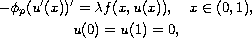where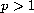(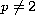),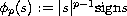,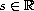,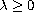, and the function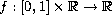is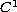and satisfies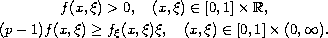These assumptions onimply that the trivial solution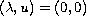is the only solution with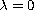or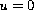, and if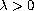then any solutionis {\em positive}, that is,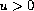on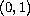.

We prove that the set of nontrivial solutions consists of acurve of positive solutions in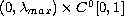, with a parametrisation of the form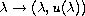, whereis afunction defined on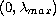, and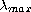is a suitable weighted eigenvalue of the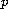-Laplacian (may be finite or), andsatisfies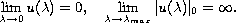We also show that for each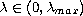the solution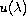is globally asymptotically stable, with respect to positive solutions (in a suitable sense).

Submitted August 13, 2009. Published April 28, 2010.
Math Subject Classifications: 34B15.
Key Words: Ordinary differential equations; p-Laplacian; nonlinear boundary value problems; positive solutions; stability.

Show me the PDF file (286 KB), TEX file, and other files for this article.Bryan P. Rynne Department of Mathematics and the Maxwell Institute for Mathematical Sciences Heriot-Watt University Edinburgh EH14 4AS, Scotland email: bryan@ma.hw.ac.uk